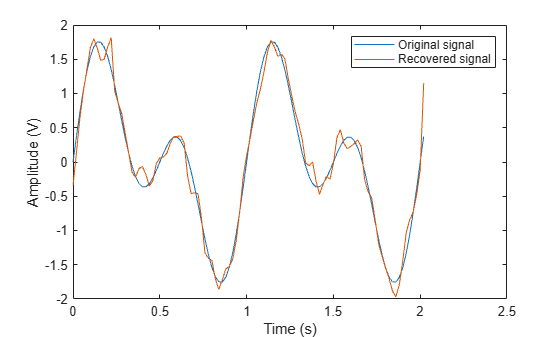Main Content

# pmdemod

Phase demodulation

## Syntax

```z = pmdemod(y,Fc,Fs,phasedev) z = pmdemod(y,Fc,Fs,phasedev,ini_phase) ```

## Description

`z = pmdemod(y,Fc,Fs,phasedev)` demodulates the phase-modulated signal `y` at the carrier frequency `Fc` (hertz). `z` and the carrier signal have sampling rate `Fs` (hertz), where `Fs` must be at least `2*Fc`. The `phasedev` argument is the phase deviation of the modulated signal, in radians.

`z = pmdemod(y,Fc,Fs,phasedev,ini_phase)` specifies the initial phase of the modulated signal, in radians.

## Examples

collapse all

Set the sample rate. To plot the signals, create a time vector.

```fs = 50; t = (0:2*fs+1)'/fs;```

Create a sinusoidal input signal.

`x = sin(2*pi*t) + sin(4*pi*t);`

Set the carrier frequency and phase deviation.

```fc = 10; phasedev = pi/2;```

Modulate the input signal.

`tx = pmmod(x,fc,fs,phasedev);`

Pass the signal through an AWGN channel.

`rx = awgn(tx,10,'measured');`

Demodulate the noisy signal.

`y = pmdemod(rx,fc,fs,phasedev);`

Plot the original and recovered signals.

```figure; plot(t,[x y]); legend('Original signal','Recovered signal'); xlabel('Time (s)') ylabel('Amplitude (V)')```## See Also

### Topics

Introduced before R2006a

## Support

#### Bridging Wireless Communications Design and Testing with MATLAB

Download white paper